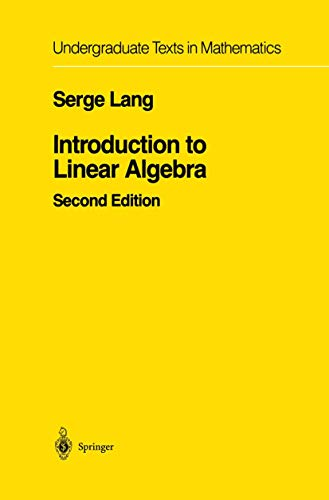• Free Shipping on all orders in Australia
• Over 7 million books in stock
• Proud to be B-Corp
• We aim to be carbon neutral by 2022
• Over 120,000 Trustpilot reviews
Item 1 of 0# Introduction to Linear Algebra by Serge Lang

Condition - New
\$113.39
Only 2 left

## Summary

This is a short text in linear algebra, intended for a one-term course. He then starts with a discussion of linear equations, matrices and Gaussian elimination, and proceeds to discuss vector spaces, linear maps, scalar products, determinants, and eigenvalues.

## Introduction to Linear Algebra Summary

### Introduction to Linear Algebra by Serge Lang

This is a short text in linear algebra, intended for a one-term course. In the first chapter, Lang discusses the relation between the geometry and the algebra underlying the subject, and gives concrete examples of the notions which appear later in the book. He then starts with a discussion of linear equations, matrices and Gaussian elimination, and proceeds to discuss vector spaces, linear maps, scalar products, determinants, and eigenvalues. The book contains a large number of exercises, some of the routine computational type, while others are conceptual.

## Introduction to Linear Algebra Reviews

Second Edition

S. Lang

Introduction to Linear Algebra

"Excellent! Rigorous yet straightforward, all answers included!"-Dr. J. Adam, Old Dominion University

I Vectors.- II Matrices and Linear Equations.- III Vector Spaces.- IV Linear Mappings.- V Composition and Inverse Mappings.- VI Scalar Products and Orthogonality.- VII Determinants.- VIII Eigenvectors and Eigenvalues.- Answers to Exercises.

NLS9781461270027
9781461270027
1461270022
Introduction to Linear Algebra by Serge Lang
New
Paperback
Springer-Verlag New York Inc.
2012-12-15
293
N/A
Book picture is for illustrative purposes only, actual binding, cover or edition may vary.
This is a new book - be the first to read this copy. With untouched pages and a perfect binding, your brand new copy is ready to be opened for the first time